Examples

Chapter 11 Class 11 Conic Sections (Term 2)
Serial order wise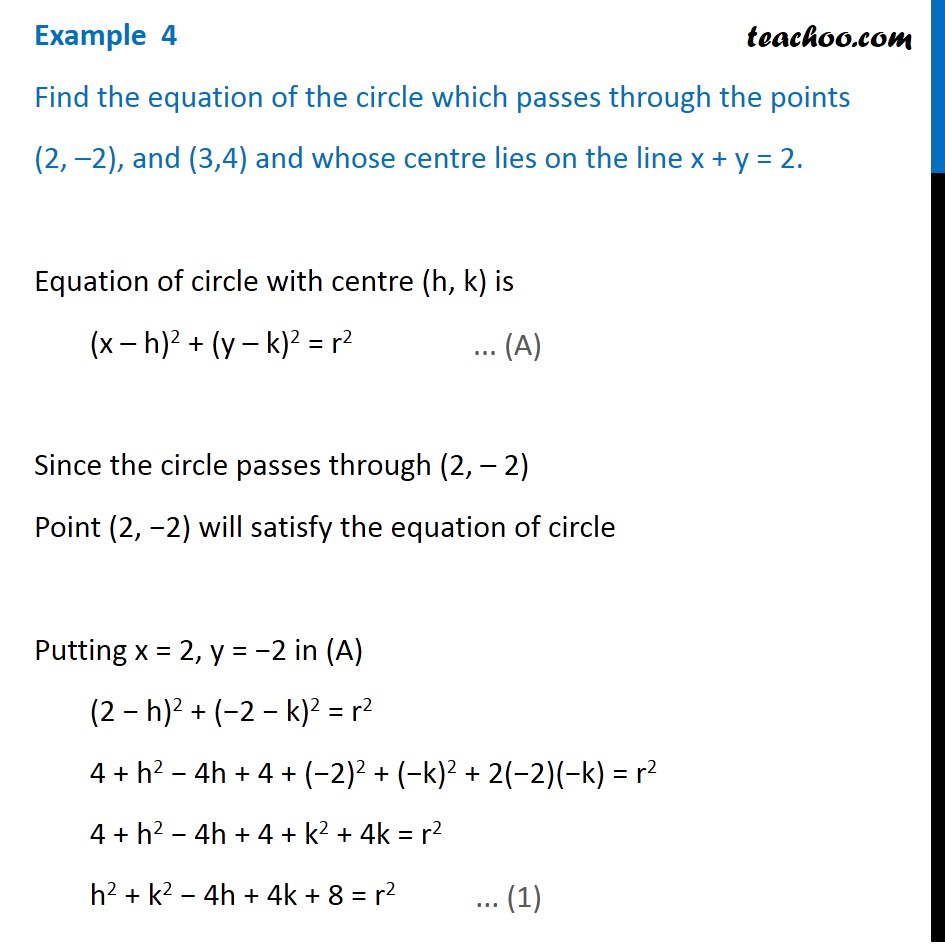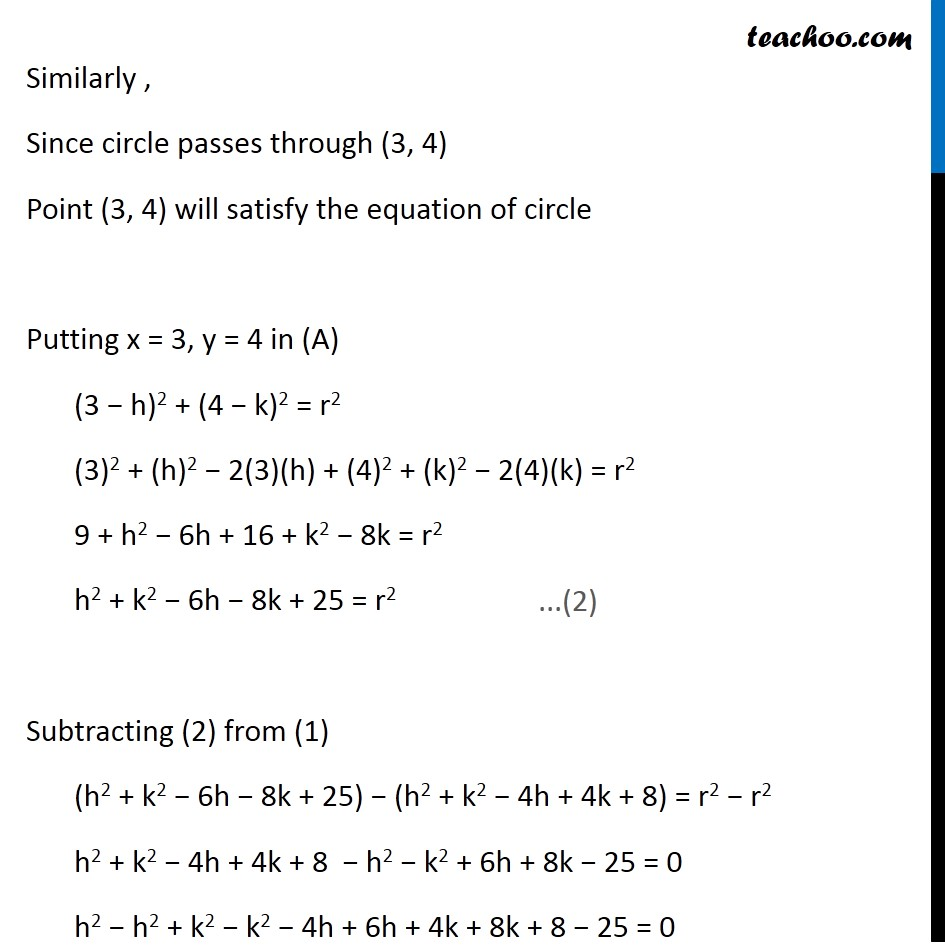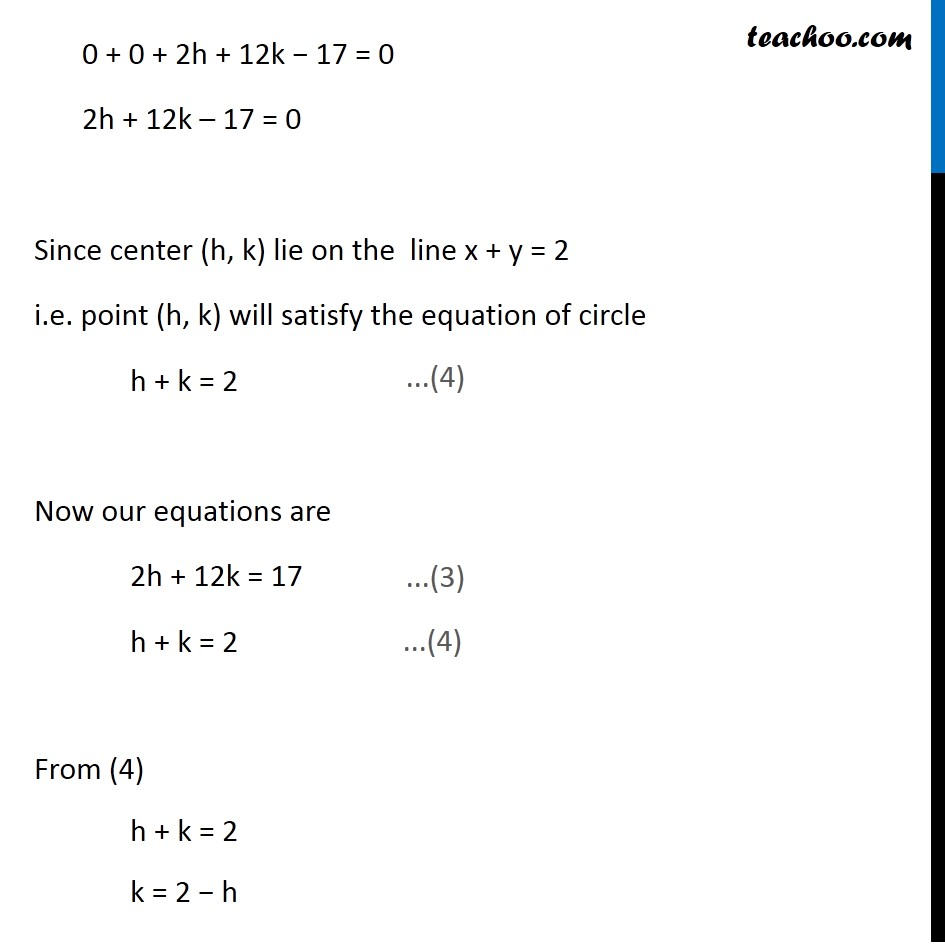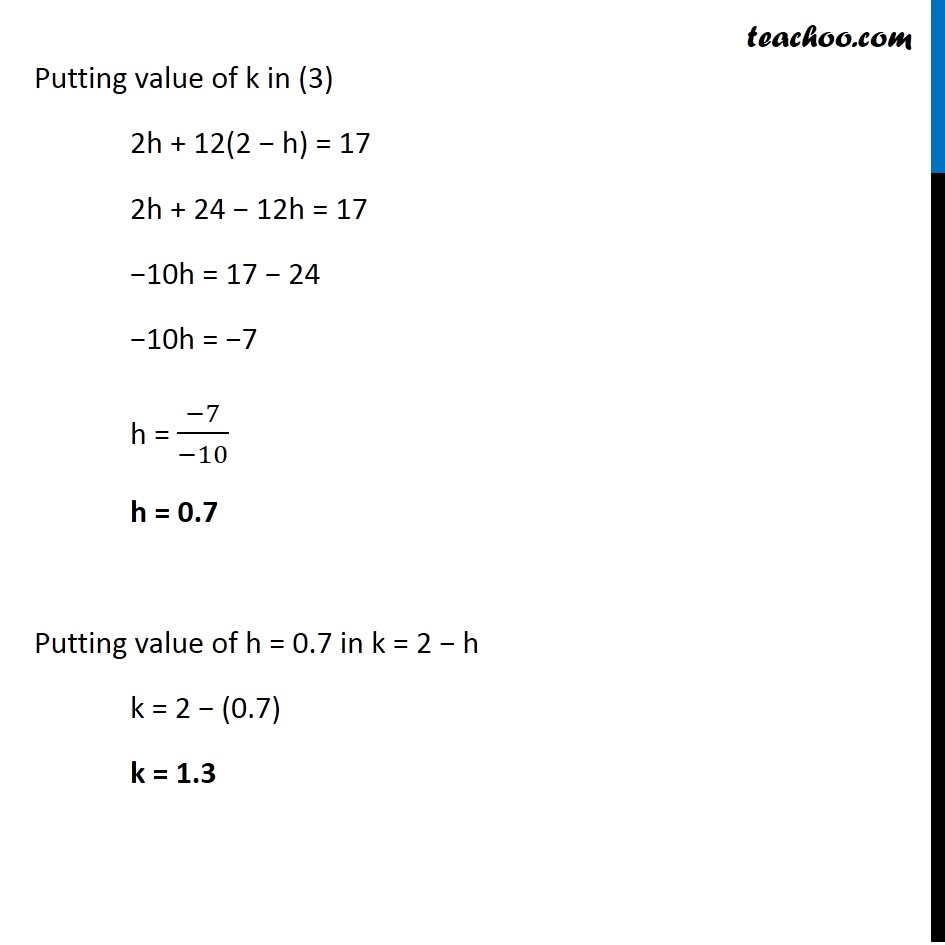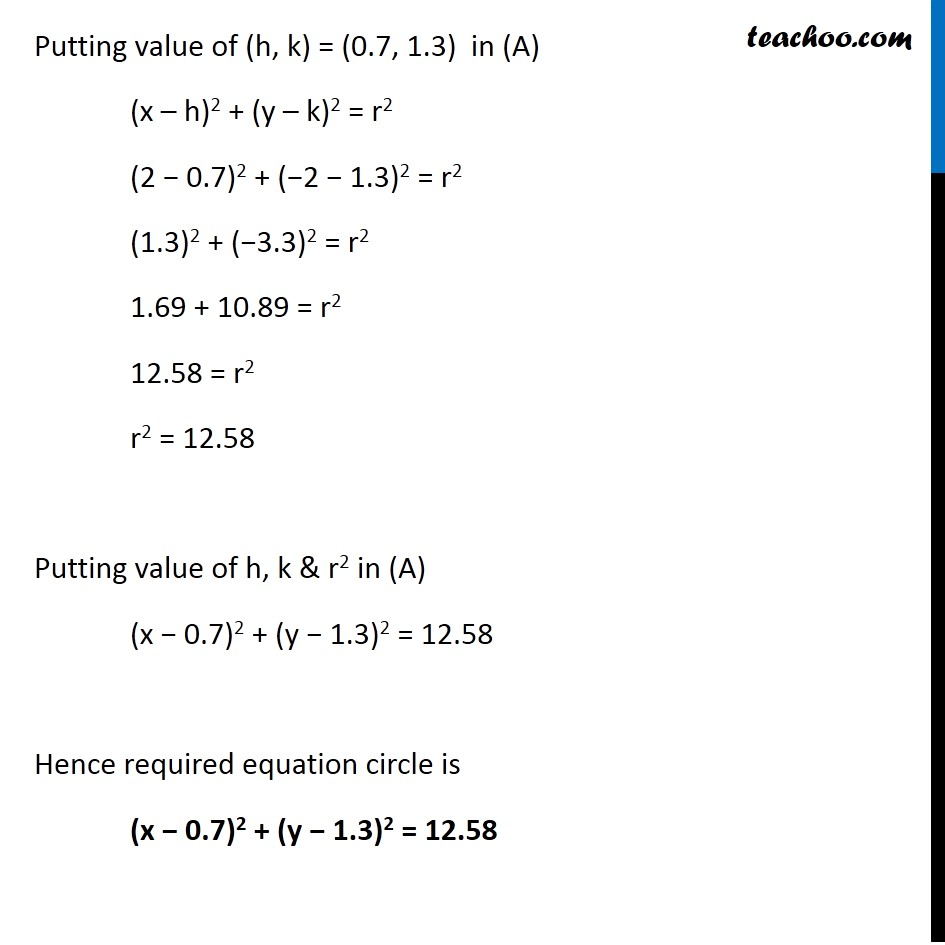### Transcript

Example 4 Find the equation of the circle which passes through the points (2, –2), and (3,4) and whose centre lies on the line x + y = 2. Equation of circle with centre (h, k) is (x – h)2 + (y – k)2 = r2 Since the circle passes through (2, – 2) Point (2, −2) will satisfy the equation of circle Putting x = 2, y = −2 in (A) (2 − h)2 + (−2 − k)2 = r2 4 + h2 − 4h + 4 + (−2)2 + (−k)2 + 2(−2)(−k) = r2 4 + h2 − 4h + 4 + k2 + 4k = r2 h2 + k2 − 4h + 4k + 8 = r2 Similarly , Since circle passes through (3, 4) Point (3, 4) will satisfy the equation of circle Putting x = 3, y = 4 in (A) (3 − h)2 + (4 − k)2 = r2 (3)2 + (h)2 − 2(3)(h) + (4)2 + (k)2 − 2(4)(k) = r2 9 + h2 − 6h + 16 + k2 − 8k = r2 h2 + k2 − 6h − 8k + 25 = r2 Subtracting (2) from (1) (h2 + k2 − 6h − 8k + 25) − (h2 + k2 − 4h + 4k + 8) = r2 − r2 h2 + k2 − 4h + 4k + 8 − h2 − k2 + 6h + 8k − 25 = 0 h2 − h2 + k2 − k2 − 4h + 6h + 4k + 8k + 8 − 25 = 0 0 + 0 + 2h + 12k − 17 = 0 2h + 12k – 17 = 0 Since center (h, k) lie on the line x + y = 2 i.e. point (h, k) will satisfy the equation of circle h + k = 2 Now our equations are 2h + 12k = 17 h + k = 2 From (4) h + k = 2 k = 2 − h Putting value of k in (3) 2h + 12(2 − h) = 17 2h + 24 − 12h = 17 −10h = 17 − 24 −10h = −7 h = (−7)/(−10) h = 0.7 Putting value of h = 0.7 in k = 2 − h k = 2 − (0.7) k = 1.3 Putting value of (h, k) = (0.7, 1.3) in (A) (x – h)2 + (y – k)2 = r2 (2 − 0.7)2 + (−2 − 1.3)2 = r2 (1.3)2 + (−3.3)2 = r2 1.69 + 10.89 = r2 12.58 = r2 r2 = 12.58 Putting value of h, k & r2 in (A) (x − 0.7)2 + (y − 1.3)2 = 12.58 Hence required equation circle is (x − 0.7)2 + (y − 1.3)2 = 12.58